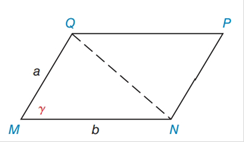Chapter 11.4, Problem 42EElementary Geometry For College St...

7th Edition
Alexander + 2 others
ISBN: 9781337614085

Solutions

Chapter
SectionElementary Geometry For College St...

7th Edition
Alexander + 2 others
ISBN: 9781337614085
Textbook Problem

Find the area of ▱ M N P Q  if  a = 8  cm,  b = 12  cm, and  γ = 70 ∘ . Answer to the nearest tenth of a square centimeter. (See Exercise 41.)Exercises 41 − 44

To determine

To find:

The area of the MNPQ

If a=8cm, b=12cm, and γ=70

Explanation

1) Formula:

The area of the parallelogram is given by,

A=absinγ unit2.

Where, a is the measure of a sides, and b is the base of the triangle,

γ is the angle between the base and the side.

2) Calculation:

Given,

The area of the MNPQ is given by,

A=absinγ unit2

Here, a=8cm, b=12cm, and γ=70

A=(8)(12

Still sussing out bartleby?

Check out a sample textbook solution.

See a sample solution

The Solution to Your Study Problems

Bartleby provides explanations to thousands of textbook problems written by our experts, many with advanced degrees!

Get Started

Evaluate the integrals in Problems 1-26.

Mathematical Applications for the Management, Life, and Social Sciences

The difference between population variance and sample variance.

Statistics for The Behavioral Sciences (MindTap Course List)

In Exercises 516, evaluate the given quantity. log1616

Finite Mathematics and Applied Calculus (MindTap Course List)

In Exercises 1-6, simplify the expression. 2. 2a23ab9b22ab2+3b3

Applied Calculus for the Managerial, Life, and Social Sciences: A Brief Approach

Factor completely. 1x2

Trigonometry (MindTap Course List)

Sometimes, Always, or Never: If for all n and both {an} and {cn} converge, then{bn} converges.

Study Guide for Stewart's Single Variable Calculus: Early Transcendentals, 8th